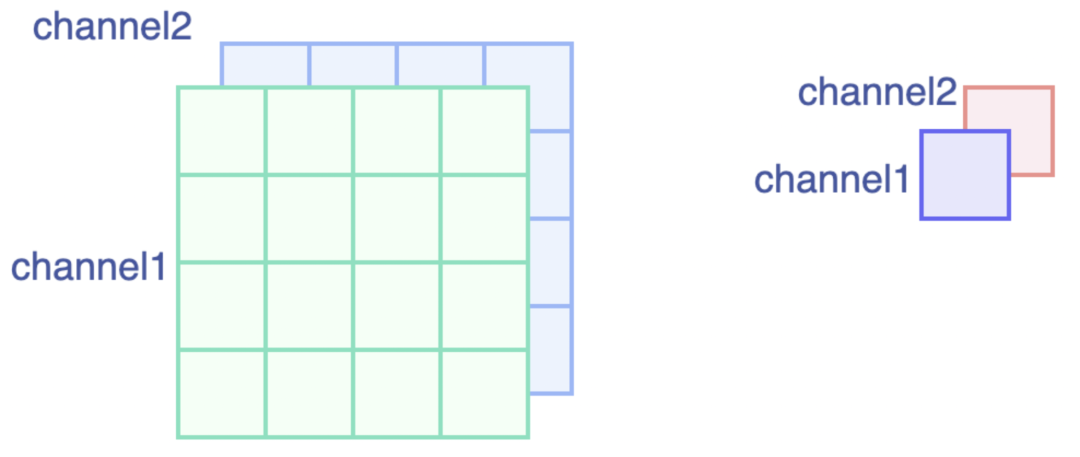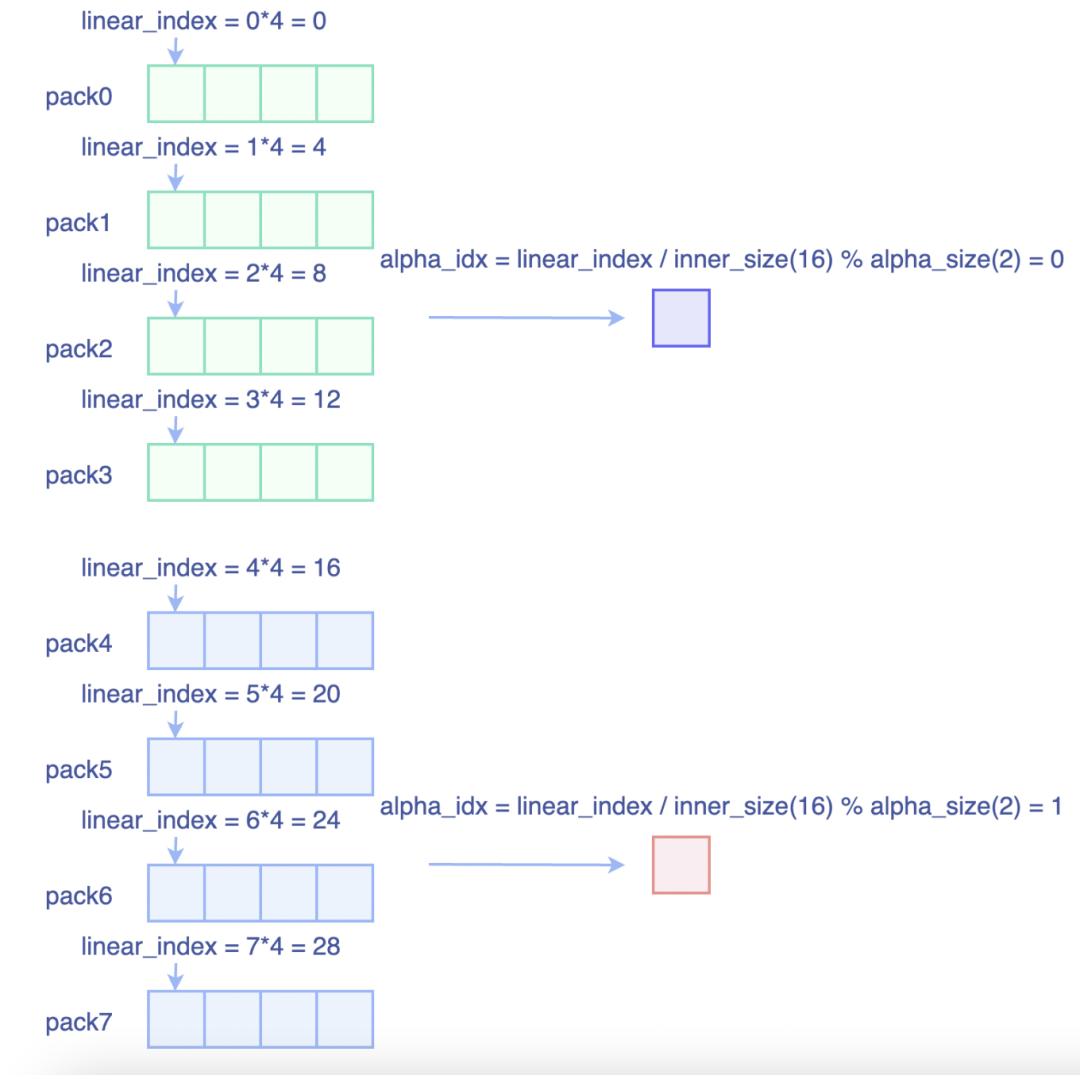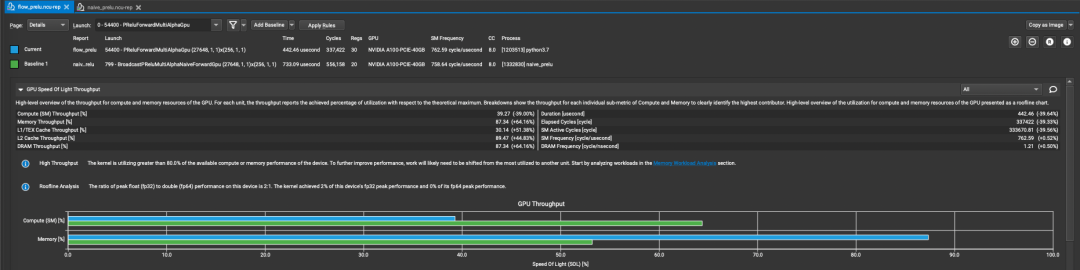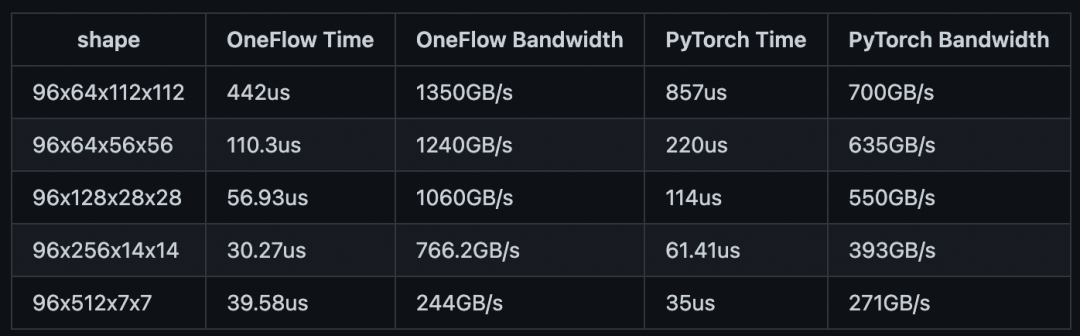# CUDA優化之PReLU效能調優

InsightFace模型裡大量使用了PReLU啟用函式，而PReLU的工作模式有兩種：

1. PReLU(1)，此時權重alpha的形狀為(1, )，等價於一個Elementwise操作。

2. PReLU(channels)，此時權重alpha的形狀為(channels, )，和輸入特徵(N, C, H, W)中C的大小是對應的。此時PReLU等價於一個Binary Broadcast操作。

1

# 樸素實現

```template<typename T> __global__ void PReluForwardGpu(const int32_t elem_cnt, const int32_t alpha_size, const int32_t inner_size, const T* x, const T* alpha, T* y) { CUDA_1D_KERNEL_LOOP(i, elem_cnt) { const T x_i = x[i]; const T alpha_i = alpha[(i / inner_size) % alpha_size]; y[i] = x_i > 0 ? x_i : x_i * alpha_i; } }```

• `inner_size`表示的是通道維後面維度乘積，以`NCHW`格式為例，`inner_size=H*W`
• `alpha_size`表示通道維大小

Integer division and modulo operation are costly as they compile to up to 20 instructions.

2

# Pack向量化優化``` template global void PReluForwardMultiAlphaGpu(const IndexType elem_cnt, const IndexType alpha_size, const IndexType inner_size, const T x, const T alpha, T* y) { int32_t global_thread_id = blockIdx.x * blockDim.x + threadIdx.x;

using LoadType = cuda::elementwise::PackType; using LoadPack = cuda::elementwise::Pack; T zero_val = static_cast(0); for (int64_t linear_index = global_thread_id * pack_size; linear_index < elem_cnt; linear_index += gridDim.x * blockDim.x * pack_size) { // 計算當前Pack所使用到Alpha的索引

``````IndexType alpha_idx = (linear_index/inner_size%alpha_size);
// 以向量化的形式載入輸入x

// 迴圈展開，逐個處理Pack內的元素
``````

# pragma unroll

``````for (int i = 0; i < pack_size; i++) {
y_vec.elem[i] = x_vec.elem[i] > zero_val ? x_vec.elem[i] : x_vec.elem[i] * alpha[alpha_idx];
}
// 以向量化的形式儲存輸出y
``````

} } ```3

# 基準測試